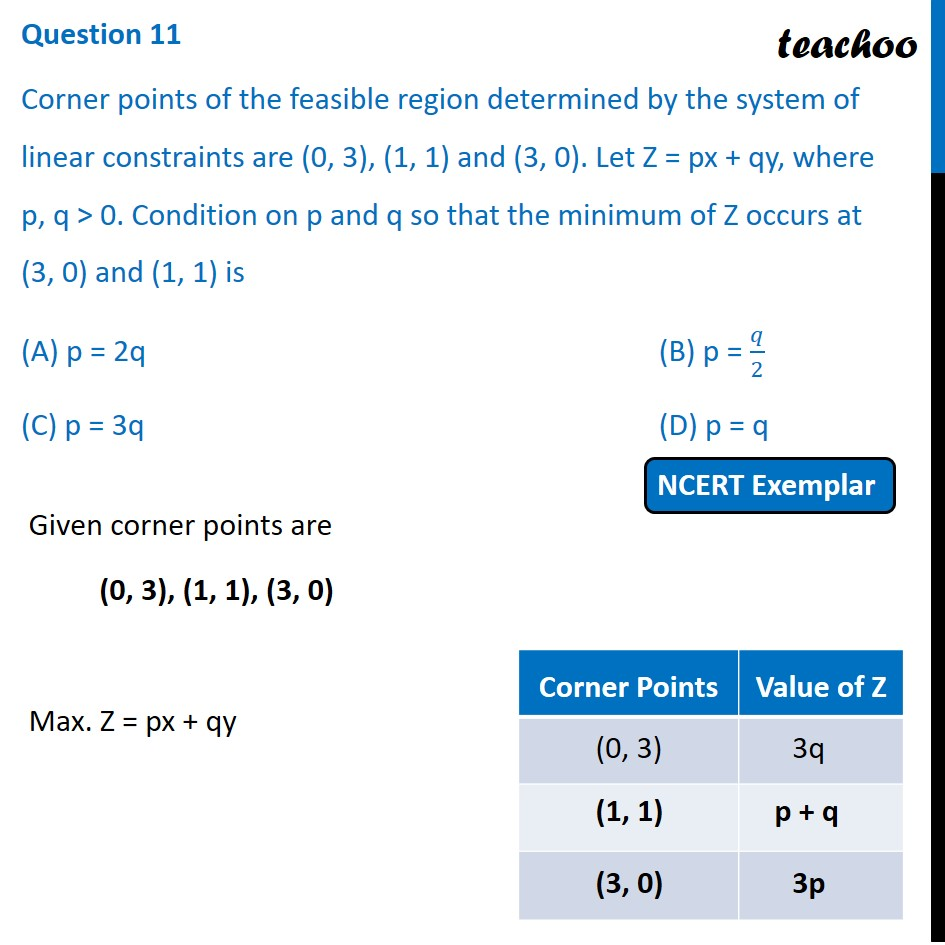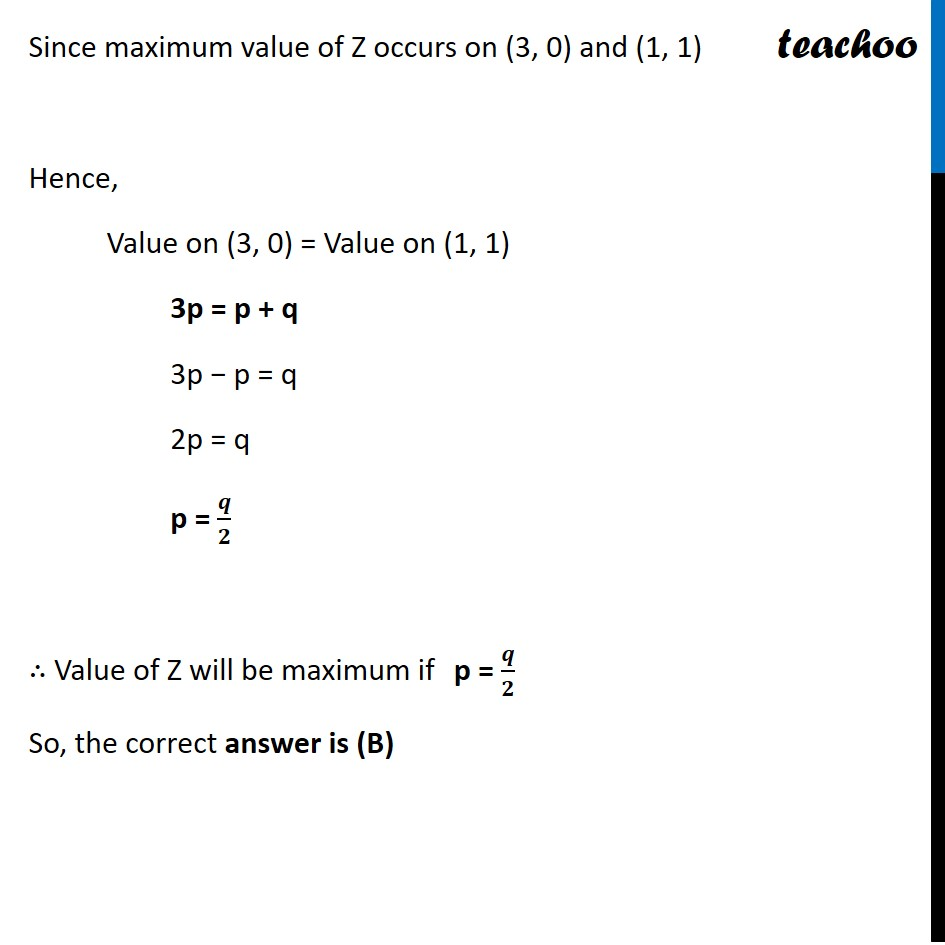NCERT Exemplar - MCQs

Chapter 12 Class 12 Linear Programming
Serial order wiseLearn in your speed, with individual attention - Teachoo Maths 1-on-1 Class

### Transcript

Question 11 Corner points of the feasible region determined by the system of linear constraints are (0, 3), (1, 1) and (3, 0). Let Z = px + qy, where p, q > 0. Condition on p and q so that the minimum of Z occurs at (3, 0) and (1, 1) is (A) p = 2q (B) p = 𝑞/2 (C) p = 3q (D) p = q Given corner points are (0, 3), (1, 1), (3, 0) Max. Z = px + qy Since maximum value of Z occurs on (3, 0) and (1, 1) Hence, Value on (3, 0) = Value on (1, 1) 3p = p + q 3p − p = q 2p = q p = 𝒒/𝟐 ∴ Value of Z will be maximum if p = 𝒒/𝟐 So, the correct answer is (B)